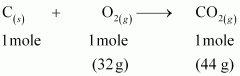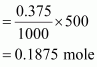# Calculate the amount of carbon dioxide that could be produced when(i) 1 mole of carbon is burnt in air.(ii) 1 mole of carbon is burnt in 16 g of dioxygen.(iii) 2 moles of carbon are burnt in 16 g of dioxygen.

10 years ago

The balanced reaction of combustion of carbon can be written as:(i) As per the balanced equation, 1 mole of carbon burns in1 mole of dioxygen (air) to produce1 mole of carbon dioxide.

(ii) According to the question, only 16 g of dioxygen is available. Hence, it will react with 0.5 mole of carbon to give 22 g of carbon dioxide. Hence, it is a limiting reactant.

(iii) According to the question, only 16 g of dioxygen is available. It is a limiting reactant. Thus, 16 g of dioxygen can combine with only 0.5 mole of carbon to give 22 g of carbon dioxide.

Questions 1.5
0.375 M aqueous solution of sodium acetate

≡ 1000 mL of solution containing 0.375 moles of sodium acetate

∴Number of moles of sodium acetate in 500 mLMolar mass of sodium acetate = 82.0245 g mole–1 (Given)

∴ Required mass of sodium acetate = (82.0245 g mol–1) (0.1875 mole)

= 15.38 g